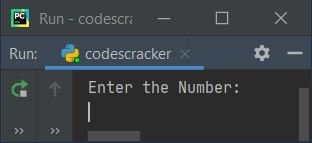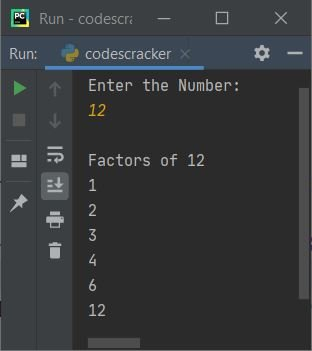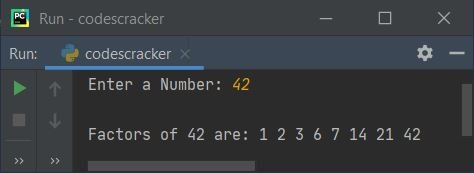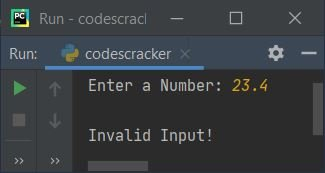# Python Program to Find Factors of a Number

This article is created to cover some programs in Python, to find and print factors of a number entered by user at run-time. Here are the list of approaches used:

• Find Factors of a Number using while Loop
• Using for Loop
• Using Function

Note - Factors of a number say n are numbers that divides the number (n) exactly. For example, factors of 12 are 1, 2, 3, 4, 6, 12. All these six numbers divides 12 without leaving any remainder.

## Find Factors of a Number using while Loop

To find factors of any number in Python, you have to ask from user to enter a number, then find and print its factors as shown in the program given below. The question is, write a Python program to find factors of a number using while loop. Here is its answer:

```print("Enter the Number: ")
num = input()

num = int(num)
print("\nFactors of", num)

i = 1
while i<=num:
if num%i==0:
print(i)
i = i+1```

Here is the initial output produced by this Python program:Now supply the input say 12 and press `ENTER` key to find and print all factors of 12 as shown in the snapshot given below:The dry run of above program with user input 12, goes like:

• Initial value, num = "12" (entered by user). In Python, anything received using input() treated as a string type value. Therefore using the following statement:
`num = int(num)`
the value of num gets converted into an integer type value. So num=12, and i=1
• Now the condition (of while loop) i<=num or 1<=12 evaluates to be true, therefore program flow goes inside the loop
• Inside the loop, the condition (of if) num%i==0 or 12%1==0 or 0==0 evaluates to be true, therefore program flow goes inside this if's body and the value of i gets printed as a factor of given number
• Now the value of i gets incremented by 1. So i=2 now
• Again the condition of while loop gets evaluated. That is the condition i<=num or 2<=12 evaluates to be true again, therefore program flow again goes inside the loop
• Inside the loop, the condition (of if) num%i==0 or 12%2==0 or 0==0 again evaluates to be true, therefore program flow again goes inside its body and the value of i gets printed as another factor of 12
• The value of i gets incremented. So i=3. Now the condition of while loop again gets evaluated. This time also the condition evaluates to be true, therefore program flow goes inside the loop
• This process continues until the condition evaluates to be false
• In this way, all factors of 12 (given number by user) gets printed, one by one

#### Modified Version of Previous Program

This is the modified version of previous program. This program uses end to skip inserting an automatic newline using print(). The str() is used to convert value to a string type. The try-except is used to handle invalid input.

```print("Enter a Number: ", end="")
try:
num = int(input())

print("\nFactors of " +str(num)+ " are: ", end="")
i = 1
while i<=num:
if num % i == 0:
print(i, end=" ")
i = i + 1
print()
except ValueError:
print("\nInvalid Input!")```

Here is its sample run with user input 42:Here is another sample run with an invalid user input say 23.4:## Find Factors of a Number using for Loop

This program does the same job as of previous program, but this program is created using for loop, instead of while. Let's have a look at the program first:

```print("Enter a Number: ", end="")
try:
num = int(input())

print("\nFactors of " +str(num)+ " are: ", end="")
for i in range(1, num+1):
if num % i == 0:
print(i, end=" ")
print()
except ValueError:
print("\nInvalid Input!")```

In above program, the following code:

`for i in range(1, num+1):`

states that the statement inside it, gets executed num number of times with value of i from 1 to num. For example, if the value of num is 12, then the loop gets evaluated 12 times with value of i from 1 to 12.

## Find Factors of a Number using Function

This program is created using a user-defined function named FindFact(). This function receives the number entered by user as its argument and prints all its factors from inside this function.

```def FindFact(n):
for i in range(1, n+1):
if n % i == 0:
print(i, end=" ")
print()

print("Enter a Number: ", end="")
try:
num = int(input())
print("\nFactors of " +str(num)+ " are: ", end="")
FindFact(num)
except ValueError:
print("\nInvalid Input!")```

This program produces exactly same output as of previous program.

## Find Factors of a Number using Class

This is the last program, created using class, an object-oriented feature of Python.

```class CodesCracker:
def FindFact(self, n):
for i in range(1, n+1):
if n % i == 0:
print(i, end=" ")

print("Enter a Number: ", end="")
try:
num = int(input())
print("\nFactors of " +str(num)+ " are: ", end="")
ob = CodesCracker()
ob.FindFact(num)
print()
except ValueError:
print("\nInvalid Input!")```

To access member function named FindFact() of a class named CodesCracker, an object of this class is required. Therefore an object ob is created of class CodesCracker, and using . (dot) operator, I've accessed the member function of the class as shown in the program given above.

Python Online Test

« Previous Program Next Program »